Display Parameters
 Show Background Animation Text Colors Basic Blue Static Spectral Colors Rotating Spectral Colors2016 Detailed List of Lecture Topics

# Lecture 1.  1st axioms and theorems of classical mechanics (8.22.16)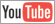(Ch. 1 thru Ch. 3 of Unit 1)

Geometry of momentum conservation axiom (ala Occam’s Razor)

Totally Inelastic “ka-runch”collisions* (begin 4:1 graph project)

Perfectly Elastic “ka-bong” and Center Of Momentum (COM) symmetry*

+Intro to weighty-averages and vector notation

Comments on idealization in classical models

Geometry of Galilean translation symmetry

45° shift in (V1,V2)-space

Time reversal symmetry

... of COM collisions

Algebra,Geometry, and Physics of momentum conservation axiom

Vector algebra of collisions

Matrix or tensor algebra of collisions

Deriving Energy Conservation Theorem

Numerical details of collision tensor algebra

Link to Main Classical Mechanics with a Bang! Web Site       Link → http://www.uark.edu/ua/modphys/markup/CMwBangWeb.html
* Launch Vehicle Collision Simulator       Link → http://www.uark.edu/ua/modphys/markup/CMMotionWeb.html
* Launch Superball Collision Simulator       Link → http://www.uark.edu/ua/modphys/markup/BounceItWeb.html

# Lecture 2.  Analysis of 1D 2-Body Collisions (8/25/2016)(Ch. 2 to Ch. 4 of Unit 1)

Review: of elastic Kinetic Energy ellipse geometry

The X2 Superball pen launcher

Perfectly elastic “ka-bong” velocity amplification effects (Faux-Flubber)

Geometry of X2 launcher bouncing in box (gravity-free)

Independent Bounce Model (IBM)

Geometric optimization and range-of-motion calculation(s)

Integration of (V1,V2) data to space-time plots (y1(t),t) and (y2(t),t) plots

Integration of (V1,V2) data to space-space plots (y1, y2) Examples (M1=7, M2=1) and (M1=49, M2=1)

Multiple collisions calculated by matrix operator products

Matrix or tensor algebra of 1-D 2-body collisions

Ellipse rescaling-geometry and reflection-symmetry analysis

Rescaling KE ellipse to circle

How this relates to Lagrangian, l’Etrangian, and Hamiltonian mechanics in Ch. 12

Reflections in the clothing store: “It’s all done with mirrors!”

Introducing hexagonal symmetry D6~C6v (Resulting for m1/m2=3)

Group multiplication and product table

Classical collision paths with D6~C6v (Resulting from m1/m2=3)

Other not-so-symmetric examples: m1/m2=4 and m1/m2=7 and (M1=100, M2=1)

Link to Main Classical Mechanics with a Bang! Web Site       Link → http://www.uark.edu/ua/modphys/markup/CMwBangWeb.html
Launch Superball Collision Simulator       Link → http://www.uark.edu/ua/modphys/markup/BounceItWeb.html
Velocity Amplification in Collision Experiments Involving Superballs - Harter et. al., Am. J. Phys. 1971
Launch Matrix Collision Simulator Under Construction!       Link → http://www.uark.edu/ua/modphys/markup/BounceMatWeb.html

# Lecture 3.  Analysis of 1D 2-Body Collisions II: Reflection groups (8/30/2016)(Ch. 2 to Ch. 4 of Unit 1)

Review: Geometry of 1-D 2-body collisions (Example with masses: m1=49 and m2=1)

Multiple collisions calculated by matrix operator products

Matrix or tensor algebra of 1-D 2-body collisions

“Mass-bang” matrix M, “Floor-bang” matrix F, “Ceiling-bang” matrix C.

Geometry and Algebra of “ellipse-Rotation” group product: R = CM

Ellipse rescaling-geometry and reflection-symmetry analysis

Rescaling KE ellipse to circle

How this relates to Lagrangian, l’Etrangian, and Hamiltonian mechanics later on

Reflections in the clothing store: “It’s all done with mirrors!”

Introducing hexagonal symmetry D6~C6v (Resulting for m1/m2=3)

Group multiplication and product table

Classical collision paths with D6~C6v (Resulting for m1/m2=3)

Other not-so-symmetric examples: m1/m2=4 and m1/m2=7 and (M1=100, M2=1)

# Lecture 4.  Kinetic Derivation of 1D Potentials and Force Fields (9/1/2016)(Ch. 6, and Ch. 7 of Unit 1)

Review of (V1, V2)→(y1, y2) relations.      High mass ratio M1/m2 = 49

Force “field” or “pressure” due to many small bounces

Force defined as momentum transfer rate

The 1D-Isothermal force field F(y)=const./y and the 1D-Adiabatic force field F(y)=const./y3

Potential field due to many small bounces

Physicist’s Definition F=-ΔU/Δy     vs.     Mathematician’s Definition F=+ΔU/Δy

Example of 1D-Isothermal potential U(y)=const. ln(y)

“Monster Mash”classical segue to Heisenberg action relations

Example of very very large M1 ball-wall(s) crushing a poor little m2

How m2 keeps its action

An interesting wave analogy: The “Tiny-Big-Bang”

A lesson in geometry of fractions and fractals: Ford Circles and Farey Sums

Lester. R. Ford, "Fractions", Am. Math. Monthly 45,586(1938)
John Farey, "On a curious Property of vulgar Fractions", Phil. Mag.(1816)
Wolfram
A. Bogomolny, Farey Series. A story from Interactive Mathematics Miscellany and Puzzles
Li, Harter, Chem.Phys.Letters (2015

# Lecture 5.  Dynamics of Potentials and Force Fields (9/6/2016)(Ch. 7 and part of Ch. 8 of Unit 1)

Potential energy geometry of Superballs and related things

Thales geometry and “Sagittal approximation” to superball force law

Geometry and dynamics of single ball bounce

(a) Constant force F=-k (linear potential V=kx )

Some physics of dare-devil diving 80 ft. into kidee pool

(b) Linear force F=-kx (quadratic potential V=½kx2 (like balloon))

(c) Non-linear force (like superball-floor or ball-bearing-anvil)

Geometry and potential dynamics of 2-ball bounce

A parable of RumpCo. vs CrapCorp. (introducing 3-mass potential-driven dynamics)

A story of USC pre-meds visiting Whammo Manufacturing Co.

Geometry and dynamics of n-ball bounces

Analogy with shockwave and acoustical horn amplifier

Advantages of a geometric m1, m2, m3,... series

A story of Stirling Colgate (Palmolive) and core-collapse supernovae

Many-body 1D collisions

Elastic examples: Western buckboard

Inelastic examples: “Zig-zag geometry” of freeway crashes

Super-elastic examples: This really is “Rocket-Science”

# Lecture 6.  Geometry of common power-law potentials (9/8/2016)(Ch. 9 of Unit 1)

Geometry of common power-law potentials

Geometric (Power) series

“Zig-Zag” exponential geometry

Projective or perspective geometry

Parabolic geometry of harmonic oscillator kr2/2 potential and -kr1 force fields

Coulomb geometry of -1/r-potential and -1/r2-force fields

Compare mks units of Coulomb Electrostatic vs. Gravity

Geometry of idealized “Sophomore-physics Earth”

Coulomb field outside Isotropic & Harmonic Oscillator (IHO) field inside

Contact-geometry of potential curve(s)

“Crushed-Earth” models: 3 key energy “steps” and 4 key energy “levels”

Earth matter vs nuclear matter:

Introducing the “neutron starlet” and  “Black-Hole-Earth”

Introducing 2D IHO orbits and phasor geometry

Phasor “clock” geometry

# Lecture 7.  Kepler Geometry of IHO (Isotropic Harmonic Oscillator) Elliptical Orbits (9/13/2016)(Ch. 9 and Ch. 11 of Unit 1)

Constructing 2D IHO orbital phasor “clock” dynamics in uniform-body

Constructing 2D IHO orbits using Kepler anomaly plots

Mean-anomaly and eccentric-anomaly geometry

Calculus and vector geometry of IHO orbits

A confusing introduction to Coriolis-centrifugal force geometry     (Derived better in Ch. 12)

Some Kepler’s “laws” for all central (isotropic) force F(r) fields

Angular momentum invariance of IHO: F(r)=-k·r with U(r)=k·r2/2      (Derived here)

Angular momentum invariance of Coulomb: F(r)=-GMm/r2 with U(r)=-GMm·/r     (Derived in Unit 5)

Total energy E=KE+PE invariance of IHO: F(r)=-k·r     (Derived here)

Total energy E=KE+PE invariance of Coulomb: F(r)=-GMm/r2     (Derived in Unit 5)

Introduction to dual matrix operator contact geometry (based on IHO orbits)

Quadratic form ellipse r•Q•r=1 vs.inverse form ellipse p•Q-1•p=1

Duality norm relations (rp=1)

Q-Ellipse tangents r′ normal to dual Q-1-ellipse position p (r′p=0=rp′)

Operator geometric sequences and eigenvectors

Alternative scaling of matrix operator geometry

Vector calculus of tensor operation

Q: Where is this headed?   A: Lagrangian-Hamiltonian duality

# Lecture 8.  Quadratic form geometry and development of mechanics of Lagrange and Hamilton (9/20/2016)(Ch. 12 of Unit 1 and Ch. 4-5 of Unit 7)

Review of partial differential calculus

Chain rule and order ∂2Ψ/∂x∂y = ∂2Ψ/∂y∂x symmetry

Scaling transformation between Lagrangian and Hamiltonian views of KE

Introducing 0th Lagrange and 0th Hamilton differential equations of mechanics

Introducing 1st Lagrange and 1st Hamilton differential equations of mechanics

Introducing the Poincare´ and Legendre contact transformations

Geometry of Legendre contact transformation (Preview of Unit 8 relativistic quantum mechanics)

Example from thermodynamics

Legendre transform: special case of General Contact Transformation (Lights, Camera, ... ACTION!)

An elementary contact transformation from sophomore physics

Algebra-calculus development of “The Volcanoes of Io” and “The Atoms of NIST”

Intuitive-geometric development of ” ” ” and ” ” ”

# Lecture 9.  Equations of Lagrange and Hamilton mechanics in Generalized Curvilinear Coordinates (GCC) (9/22/2016)(Ch. 12 of Unit 1, Ch. 1-5 of Unit 2, and Ch. 1-5 of Unit 3)

Quick Review of Lagrange Relations in Lectures 8-9

Using differential chain-rules for coordinate transformations

Polar coordinate example of Generalized Curvilinear Coordinates (GCC)

Getting the GCC ready for mechanics: Generalized velocity and Jacobian Lemma 1

Getting the GCC ready for mechanics: Generalized acceleration and Lemma 2

How to say Newton’s “F=ma” in Generalized Curvilinear Coords.

Use Cartesian KE quadratic form KE=T=v•M•v/2 and F=M•a to get GCC force

Lagrange GCC trickery gives Lagrange force equations

Lagrange GCC trickery gives Lagrange potential equations (Lagrange 1 and 2)

GCC Cells, base vectors, and metric tensors

Polar coordinate examples: Covariant Em vs. Contravariant Em

Covariant metric gmn vs. Invariant δmn vs. Contravariant metric gmn

Lagrange prefers Covariant gmn with Contravariant velocity

GCC Lagrangian definition

GCC “canonical” momentum pm definition

GCC “canonical” force Fm definition/p>

Coriolis “fictitious” forces (… and weather effects)

# Lecture 10.  Hamiltonian vs. Lagrange mechanics in Generalized Curvilinear Coordinates (GCC) (9/23/2016)(Unit 1 Ch. 12, Unit 2 Ch. 2-7, Unit 3 Ch. 1-3)

Review of Lectures 9-12 procedures:

Lagrange prefers Covariant metric gmn with Contravariant velocity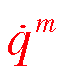Hamilton prefers Contravariant metric gmn with Covariant momentum pm

Deriving Hamilton’s equations from Lagrange’s equations

Expressing Hamiltonian H(momentum pm,qn) using gmn and covariant momentum pm

Polar-coordinate example of Hamilton’s equations

Hamilton’s equations in Runga-Kutta (computer solution) form

Examples of Hamiltonian mechanics in effective potentials

Isotropic Harmonic Oscillator in polar coordinates and effective potential

Coulomb orbits in polar coordinates and effective potential

Parabolic and 2D-IHO orbital envelopes

Clues for future assignment _

Examples of Hamiltonian mechanics in phase plots

1D Pendulum and phase plot (Web Simulations: Pendulum, Cycloidulum (Constrained Pendulum), and JerkIt (Vertically Driven Pendulum))

1D-HO phase-space control Classic simulation of “Catcher in the Eye” and new web simulation in JerkIt under developement.

# Lecture 11.  Poincare, Lagrange, Hamiltonian, and Jacobi mechanics (9/27/2016)(Unit 1 Ch. 12, Unit 2 Ch. 2-7, Unit 3 Ch. 1-3, Unit 7 Ch. 1-2)

Parabolic and 2D-IHO orbital envelopes ( Review of Lecture 9 p.56-81 and a generalization.)

Clues for future assignment

Examples of Hamiltonian mechanics in phase plots

1D Pendulum and phase plot (Web Simulations: Pendulum, Cycloidulum (Constrained Pendulum), and JerkIt (Vertically Driven Pendulum))

1D-HO phase-space control (Old Mac OS and Web Simulation of “Catcher in the Eye”)

Exploring phase space and Lagrangian mechanics more deeply

A weird “derivation” of Lagrange’s equations

Poincare identity and Action, Jacobi-Hamilton equations

How Classicists might have “derived” quantum equations

Huygen’s contact transformations enforce minimum action

How to do quantum mechanics if you only know classical mechanics

(“Color-Quantization” simulations: Davis-Heller “Color-Quantization” or “Classical Chromodynamics”)

# Lecture 12.  Complex Variables, Series, and Field Coordinates I. (9/29/2016)(Ch. 10 of Unit 1)

 1. The Story of e (A Tale of Great \$Interest\$) How good are those power series? Taylor-Maclaurin series, imaginary interest, and complex exponentials 2. What good are complex exponentials? Easy trig    Easy 2D vector analysis       Easy oscillator phase analysis          Easy rotation and “dot” or “cross” products 3. Easy 2D vector calculus    Easy 2D vector derivatives    Easy 2D source-free field theory       Easy 2D vector field-potential theory 4. Riemann-Cauchy relations (What’s analytic? What’s not?) Easy 2D curvilinear coordinate discovery Easy 2D circulation and flux integrals    Easy 2D monopole, dipole, and 2n-pole analysis       Easy 2n-multipole field and potential expansion          Easy stereo-projection visualization             Cauchy integrals, Laurent-Maclaurin series 5. Mapping and Non-analytic 2D source field analysis 1. Complex numbers provide "automatic trigonometry" 2. Complex numbers add like vectors. 3. Complex exponentials Ae-iωt track position and velocity using Phasor Clock. 4. Complex products provide 2D rotation operations. 5. Complex products provide 2D “dot”(•) and “cross”(x) products. 6. Complex derivative contains “divergence”(∇•F) and “curl”(∇xF) of 2D vector field 7. Invent source-free 2D vector fields [∇•F=0 and ∇xF=0] 8. Complex potential φ contains “scalar”( F= ∇Φ) and “vector”( F=∇xA) potentials. The half-nʼ-half results: (Riemann-Cauchy Derivative Relations) 9. Complex potentials define 2D Orthogonal Curvilinear Coordinates (OCC) of field 10. Complex integrals ∫ f(z)dz count 2D “circulation”( ∫F•dr) and “flux”( ∫Fxdr) 11. Complex integrals define 2D monopole fields and potentials 12. Complex derivatives give 2D dipole fields 13. More derivatives give 2D 2N-pole fields… 14. ...and 2N-pole multipole expansions of fields and potentials... 15. ...and Laurent Series... 16. ...and non-analytic source analysis.

# Lecture 13.  Complex Variables, Series, and Field Coordinates II. (10/4/2016)(Ch. 10 of Unit 1)

 1. The Story of e (A Tale of Great \$Interest\$) How good are those power series? Taylor-Maclaurin series, imaginary interest, and complex exponentials 2. What good are complex exponentials? Easy trig    Easy 2D vector analysis       Easy oscillator phase analysis          Easy rotation and “dot” or “cross” products 3. Easy 2D vector calculus    Easy 2D vector derivatives    Easy 2D source-free field theory       Easy 2D vector field-potential theory 4. Riemann-Cauchy relations (What’s analytic? What’s not?) Easy 2D curvilinear coordinate discovery Easy 2D circulation and flux integrals    Easy 2D monopole, dipole, and 2n-pole analysis       Easy 2n-multipole field and potential expansion          Easy stereo-projection visualization             Cauchy integrals, Laurent-Maclaurin series 5. Mapping and Non-analytic 2D source field analysis 1. Complex numbers provide "automatic trigonometry" 2. Complex numbers add like vectors. 3. Complex exponentials Ae-iωt track position and velocity using Phasor Clock. 4. Complex products provide 2D rotation operations. 5. Complex products provide 2D “dot”(•) and “cross”(x) products. Lecture 16 Thur. 10.16.14 starts here ⤵ 6. Complex derivative contains “divergence”(∇•F) and “curl”(∇xF) of 2D vector field 7. Invent source-free 2D vector fields [∇•F=0 and ∇xF=0] 8. Complex potential φ contains “scalar”( F= ∇Φ) and “vector”( F=∇xA) potentials. The half-nʼ-half results: (Riemann-Cauchy Derivative Relations) 9. Complex potentials define 2D Orthogonal Curvilinear Coordinates (OCC) of field 10. Complex integrals ∫ f(z)dz count 2D “circulation”( ∫F•dr) and “flux”( ∫Fxdr) 11. Complex integrals define 2D monopole fields and potentials 12. Complex derivatives give 2D dipole fields 13. More derivatives give 2D 2N-pole fields… 14. ...and 2N-pole multipole expansions of fields and potentials... 15. ...and Laurent Series... 16. ...and non-analytic source analysis.

# Lecture 14.  Introducing GCC Lagrangian`a la Trebuchet*Dynamics *treb-yew-shay (10/6/2016) <Ch. 1-3 of Unit 2 and Unit 3 (Mostly Unit 2.)

The trebuchet (or ingenium) and its cultural relevancy (3000 BCE to 21st )

The medieval ingenium (9th to 14th century) and modern re-enactments

Human kinesthetics and sports kinesiology

Review of Lagrangian equation derivation from Lecture 10 (Now with trebuchet model)

Coordinate geometry, Jacobian, velocity, kinetic energy, and dynamic metric tensor γmn

Structure of dynamic metric tensor γmn

Basic force, work, and acceleration

Lagrangian force equation

Canonical momentum and γmn tensor

Summary of Lagrange equations and force analysis (Mostly Unit 2.)

Forces: total, genuine, potential, and/or fictitious

Geometric and topological properties of GCC transformations (Mostly from Unit 3.)

Multivalued functionality and connections

Covariant and contravariant relations

Tangent space vs. Normal space

Metric gmn tensor geometric relations to length, area, and volume

# Lecture 15.  GCC Lagrange and Riemann Equations for Trebuchet (10/11/2016)(Ch. 1-5 of Unit 2 and Unit 3)

Review (Mostly Unit 2.): Was the Trebuchet a dream problem for Galileo? Not likely.

Forces in Lagrange force equation: total, genuine, potential, and/or fictitious

Geometric and topological properties of GCC transformations (Mostly from Unit 3.)

Trebuchet Cartesian projectile coordinates are double-valued

Toroidal “rolled-up” (q1=θ, q2=φ)-manifold and “Flat” (x=θ, y=φ)-graph

Review of covariant En and contravariant Em vectors: Jacobian J vs. Kajobian K

Covariant metric gmn vs. contravariant metric gmn (Lect. 9 p.53)

Tangent {En} space vs. Normal {Em} space

Covariant vs. contravariant coordinate transformations

Metric gmn tensor geometric relations to length, area, and volume

Lagrange force equation analysis of trebuchet model (Mostly from Unit 2.)

Review of trebuchet canonical (covariant) momentum and mass metric γmn Lect. 14 p. 77)

Review and application of trebuchet covariant forces Fθ and Fφ (Lect. 14 p. 69)

Riemann equation derivation for trebuchet model

Riemann equation force analysis

2nd-guessing Riemann equation?

# Lecture 16.  Hamilton Equations for Trebuchet and Other Things (10/13/2016)(Ch. 5-9 of Unit 2)

Review of Hamiltonian equation derivation (Elementary trebuchet)

Hamiltonian definition from Lagrangian and γmn tensor

Hamilton’s equations and Poincare invariant relations

Hamiltonian expression and contravariant γmn tensor

Hamiltonian energy and momentum conservation and symmetry coordinates

Coordinate transformation helps reduce symmetric Hamiltonian

Free-space trebuchet kinematics by symmetry

Algebraic approach

Direct approach and Superball analogy

Trebuchet vs Flinger and sports kinematics

Many approaches to Mechanics

Untreated external examples of other 'simple' machines capable of very complex (chaotic) motion:
Compound pendulum at the US National Center for Atmospheric Research (NCAR) in Boulder, CO.

Triple pendulum at the University of Sydney School of Physics in AU

# Lecture 17.  Reimann-Christoffel equations and covariant derivative (10/20/2016)(Ch. 5-9 of Unit 2)

Covariant derivative and Christoffel Coefficients Γij;k and Γij;k

Christoffel g-derivative formula

What’s a tensor? What’s not?

General Riemann equations of motion (No explicit t-dependence and fixed GCC)

Riemann-forms in cylindrical polar OCC (q1 = ρ, q2 = φ, q3 = z)

Christoffel relation to Coriolis coefficients

Mechanics of ideal fluid vortex

Separation of GCC Equations: Effective Potentials

Small (nρ:mφ)-periodic and quasi-periodic oscillations

2D Spherical pendulum“Bowl-Bowling” and the “I-Ball”

(nρ:mφ)=(2:1) vs (1:1) periodic and quasi-periodic orbits

Cycloidal ruler&compass geometry

(To be applied to mechanics in electromagnetic fields and collisional rotation in following lectures.)

# Lecture 18.  Electromagnetic Lagrangian and charge-field mechanics (10/25/2016)(Ch. 2.8 of Unit 2)

 Charge mechanics in electromagnetic fields Vector analysis for particle-in-(A,Φ)-potential Lagrangian for particle-in-(A,Φ)-potential Hamiltonian for particle-in-(A,Φ)-potential Canonical momentum in (A,Φ) potential Hamiltonian formulation Hamilton’s equations Crossed E and B field mechanics Classical Hall-effect and cyclotron orbit and equations Vector theory vs. complex variable theory Mechanical analog of cyclotron and FBI rule Cycloidal ruler&compass geometry Cycloidal geometry of flying levers      Practical poolhall application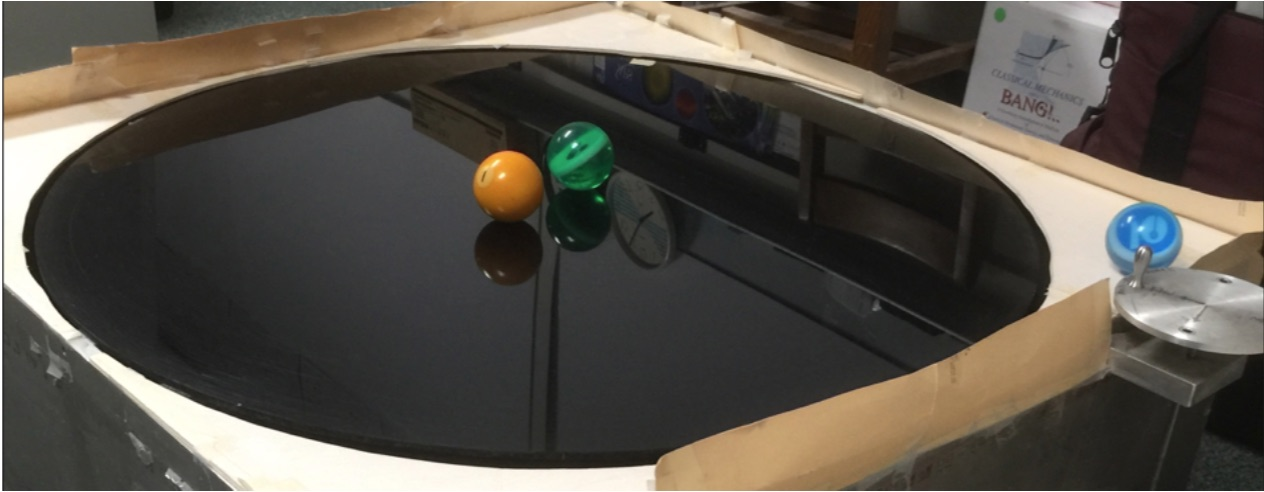This mechanical analog of (Ex,Bz) field mimics A-field with tabletop v-field# Lecture 19.  Classical Constraints: Comparing various methods (10/27/2016)(Ch. 9 of Unit 3)

Some Ways to do constraint analysis

Way 1. Simple constraint insertion

Way 2. GCC constraint webs

Find covariant force equations

Compare covariant vs. contravariant forces

Other Ways to do constraint analysis

Way 3. OCC constraint webs

Sketch of atomic-Stark orbit parabolic OCC analysis

Classical Hamiltonian separability

Way 4. Lagrange multipliers

Lagrange multiplier as eigenvalues

Multiple multipliers

“Non-Holonomic” multipliers

Cycloid-like curves for rolling constraints

# Lecture 20.  Introduction to classical oscillation and resonance (11/3/2016)(Ch. 1 of Unit 4)

1D forced-damped-harmonic oscillator equations and Green’s function solutions

Linear harmonic oscillator equation of motion.

Linear damped-harmonic oscillator equation of motion.

Frequency retardation and amplitude damping.

Figure of oscillator merit (the 5% solution 3/Γ and other numbers)

Linear forced-damped-harmonic oscillator equation of motion.

Phase lag and amplitude resonance amplification

Figure of resonance merit: Quality factor q=ω0/2Γ

Properties of Green’s function solutions and their mathematical/physical behavior

Transient solutions vs. Steady State solutions

Complete Green’s Solution for the FDHO (Forced-Damped-Harmonic Oscillator)

Quality factors: Beat, lifetimes, and uncertainty

Approximate Lorentz-Green’s Function for high quality FDHO (Quantum propagator)

Common Lorentzian (a.k.a. Witch of Agnesi)

# Lecture 21.  Introduction to coupled oscillation and eigenmodes (11/4/2016)(Ch. 2-4 of Unit 4 11.12.15)

 2D harmonic oscillator equations Lagrangian and matrix forms and Reciprocity symmetry 2D harmonic oscillator equation eigensolutions Geometric method Matrix-algebraic eigensolutions with example M =Secular equation Hamilton-Cayley equation and projectors Idempotent projectors (how eigenvalues ⇒ eigenvectors) Operator orthonormality and Completeness (Idempotent means: P·P=P) Spectral Decompositions Functional spectral decomposition Orthonormality vs. Completeness vis-a`-vis Operator vs. State Lagrange functional interpolation formula Diagonalizing Transformations (D-Ttran) from projectors 2D-HO eigensolution example with bilateral (B-Type) symmetry Mixed mode beat dynamics and fixed π/2 phase 2D-HO eigensolution example with asymmetric (A-Type) symmetry Initial state projection, mixed mode beat dynamics with variable phaseVideos of Coupled Pendula w/overhead projector for display Moderate coupling:Stronger coupling:# Lecture 22.  Introduction to Spinor-Vector resonance dynamics (11/8/2016)(Ch. 2-4 of Unit 4 Ch. 6-7 of Unit 6)

Review: 2D harmonic oscillator equations with Lagrangian and matrix forms

ANALOGY: 2-State Schrodinger: iħ∂t|Ψ(t)〉=H|Ψ(t)〉 versus Classical 2D-HO: ∂2tx=-K•x

Hamilton-Pauli spinor symmetry ( σ-expansion in ABCD-Types) H=ωμσμ

Derive σ-exponential time evolution (or revolution) operator U = e-iHt = e-iσµωµt

Spinor arithmetic like complex arithmetic

Spinor vector algebra like complex vector algebra

Spinor exponentials like complex exponentials ("Crazy-Thing"-Theorem)

Geometry of evolution (or revolution) operator U = e-iHt = e-iσµωµt

The "mysterious" factors of 2 (or 1/2): 2D Spinor vs 3D Spin Vector space

2D Spinor vs 3D vector rotation

NMR Hamiltonian: 3D Spin Moment m in B field

Euler's state definition using rotations R(α,0,0), R(0,β,0),and R(0,0,γ)

Spin-1 (3D-real vector) case

Spin-1/2 (2D-complex spinor) case

3D-real Stokes Vector defines 2D-HO polarization ellipses and spinor states

Asymmetry SA=SZ, Balance SB =SX, and Chirality SC =SY

Polarization ellipse and spinor state dynamics

The "Great Spectral Avoided-Crossing" and A-to-B-to-A symmetry breaking

# Lecture 23.  U(2)~R(3) algebra/geometry in classical or quantum theory (11/10/2016)(Classical Mechanics with a BANG! Units 4-6, Quantum Theory for Computer Age - Ch. 10A-B of Unit 3)

(Principles of Symmetry, Dynamics, and Spectroscopy - Sec. 1-3 of Ch. 5 and Ch. 7)

Reviewing fundamental Euler R(αβγ) and Darboux R[ϕϑΘ] representations of U(2) and R(3)

Euler-defined state |αβγ〉 described by Stoke’s S-vector, phasors, or ellipsometry

Darboux defined Hamiltonian H = [ϕϑΘ] = exp(-iΩ•S)·t and angular velocity Ω(φθ)·t=Θ-vector

Euler-defined operator R(αβγ) derived from Darboux-defined R[ϕϑΘ] and vice versa

Euler R(αβγ) rotation Θ =0-4π-sequence [ϕϑ] fixed (and “real-world” applications)

Quick U(2) way to find eigen-solutions for 2-by-2 Hamiltonian H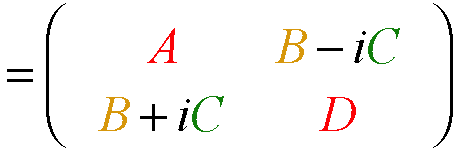The ABC’s of U(2) dynamics-Archetypes Asymmetric-Diagonal A-Type motion Bilateral-Balanced B-Type motion Circular-Coriolis… C-Type motion The ABC’s of U(2) dynamics-Mixed modes AB-Type motion and Wigner’s Avoided-Symmetry-Crossings ABC-Type elliptical polarized motion Ellipsometry using U(2) symmetry coordinates Conventional amp-phase ellipse coordinates Euler Angle (αβγ) ellipse coordinates Addenda: U(2) density matrix formalism Bloch equation for density operator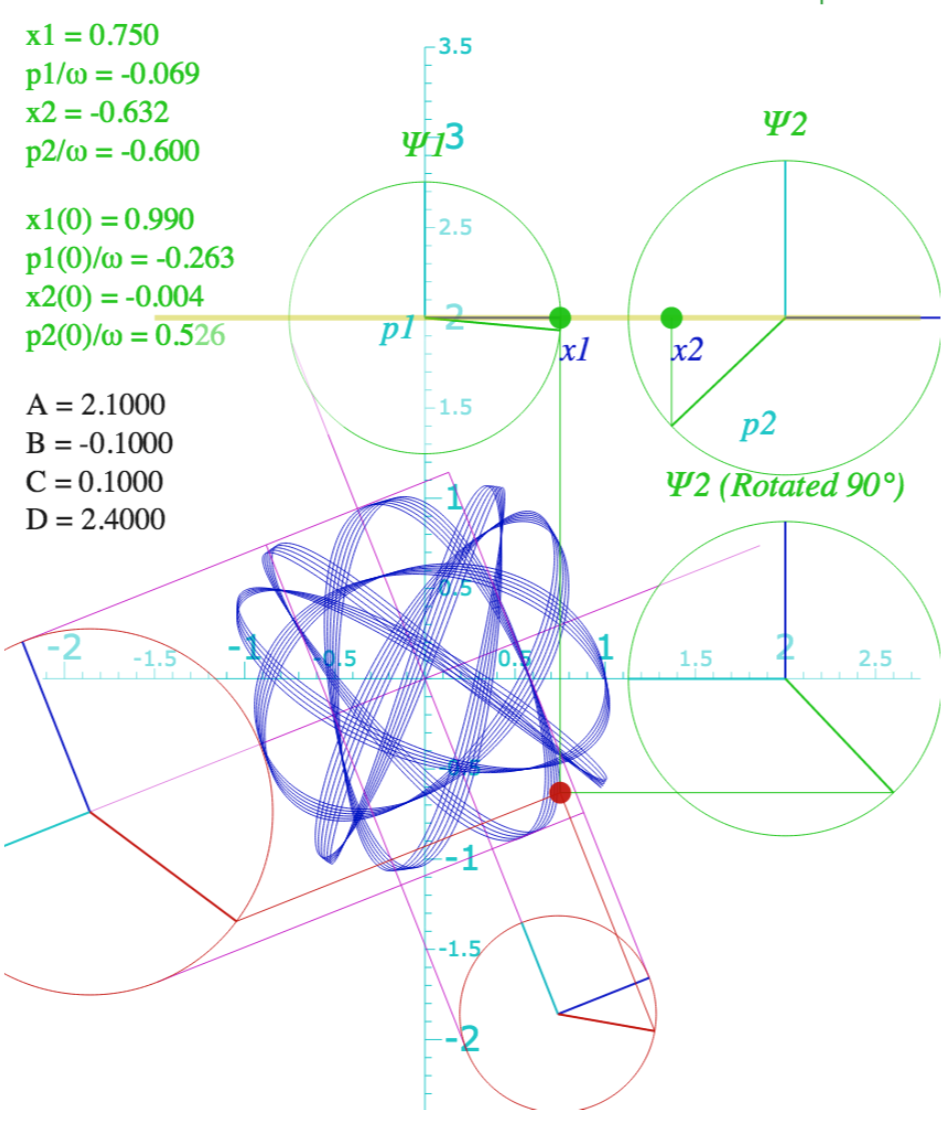# Lecture 24.  Parametric Resonance and Multi-particle Wave Modes (11/17/2016)(Ch. 7-8 of Unit 4 11.24.15)

Two Kinds of Resonance: Linear-additive vs. Nonlinear-multiplicative (Parametric resonance)

 Coupled rotation and translation (Throwing revisited: trebuchet, atlatl, etc.) Schrodinger wave equation related to Parametric resonance dynamics Electronic band theory and analogous mechanics Wave resonance in cyclic symmetry Harmonic oscillator with cyclic C2 symmetry C2 symmetric (B-type) modes Harmonic oscillator with cyclic C3 symmetry C3 symmetric spectral decomposition by 3rd roots of unity Resolving C3 projectors and moving wave modes Dispersion functions and standing waves C6 symmetric mode model:Distant neighbor coupling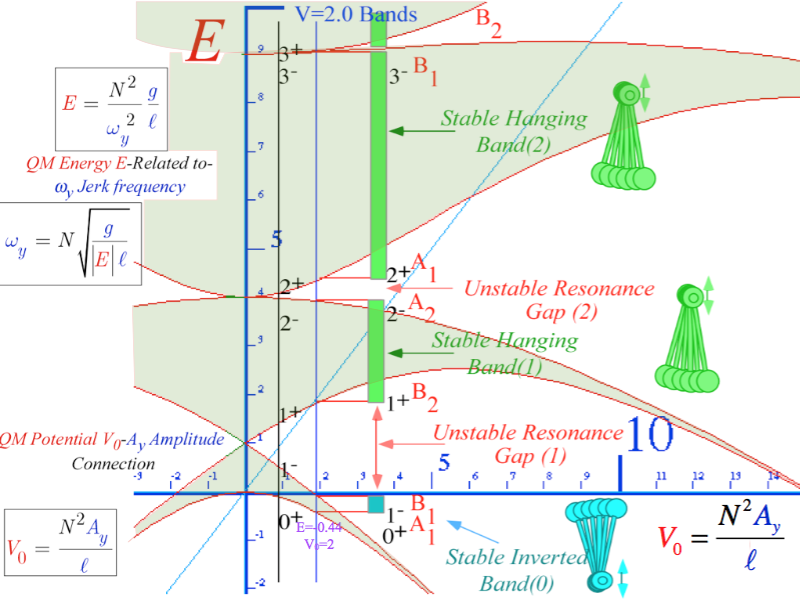C6 spectra of gauge splitting by C-type symmetry(complex, chiral, coriolis, current, ...)

CN symmetric mode models: Made-to order dispersion functions

Quadratic dispersion models: Super-beats and fractional revivals

Phase arithmetic

Algebra and geometry of resonant revivals: Farey Sums and Ford Circles

Relating CN symmetric H and K matrices to differential wave operators

# Lecture 25.  Introduction to Orbital Dynamics (11/18/2016)(Ch. 2-4 of Unit 5 12.01.15)

Orbits in Isotropic Harmonic Oscillator and Coulomb Potentials

Effective potentials for IHO and Coulomb orbits     Review: “3steps from Hell” (Lect. 7 Ch. 9 Unit 1)

Classical turning radii and apogee/perigee parameters     ← (A mysterious similarity appears)

Polar coordinate differential equations     ← (A mysterious similarity appears)

Detailed orbital functions     ← (A mysterious similarity appears)

Relating orbital energy-momentum to conic-sectional orbital geometry

Kepler equation of time and phase geometry

Geometry and Symmetry of Coulomb orbits

Detailed elliptic geometry

Detailed hyperbolic geometry

# Lecture 26.  Geometry and Symmetry of Coulomb Orbital Dynamics I. (11/22/2016)(Ch. 2-4 of Unit 5 11.22.16)

Rutherford scattering and hyperbolic orbit geometry

Backward vs forward scattering angles and orbit construction example

Parabolic “kite” and orbital envelope geometry

Differential and total scattering cross-sections

Eccentricity vector ε and (ε)-geometry of orbital mechanics

Projection ε•r geometry of ε-vector and orbital radius r

Review and connection to usual orbital algebra (previous lecture)

Projection ε•p geometry of ε-vector and momentum p=mv

General geometric orbit construction using ε-vector and (γ,R)-parameters

Derivation of ε-construction by analytic geometry

Coulomb orbit algebra of ε-vector and Kepler dynamics of momentum p=mv

Example of complete (r,p)-geometry of elliptical orbit

Connection formulas for (a,b) and (ε,λ) with (γ,R)

# Lecture 27.  Geometry and Symmetry of Coulomb Orbital Dynamics II. (11/29/2016)(Ch. 2-4 of Unit 5 12.05.15)

Review of Eccentricity vector ε and (ε,λ)-geometry of orbital mechanics    ← Review of lecture 26

Analytic geometry derivation of ε-construction    ← Review of lecture 26

Connection formulas for (a,b) and (ε,λ) with (γ,R)    ← Review of lecture 26

 Detailed ruler & compass construction of ε-vector and orbits (R = -0.375 elliptic orbit) (R = +0.5 hyperbolic orbit) Properties of Coulomb trajectory families and envelopes Graphical ε-development of orbits Launch angle fixed-Varied launch energy Launch energy fixed-Varied launch angle Launch optimization and orbit family envelopes# Lecture 28.  Multi-particle and Rotational Dynamics (11/1/2016)(Ch. 2-7 of Unit 6 12.01.16)

2-Particle orbits

Ptolemetric or LAB view and reduced mass

Copernican or COM view and reduced coupling

2-Particle orbits and scattering: LAB-vs.-COM frame views

Ruler & compass construction (or not)

Rotational equivalent of Newton’s F=dp/dt equations: N=dL/dt

How to make my boomerang come back

The gyrocompass and mechanical spin analogy

Rotational momentum and velocity tensor relations

Quadratic form geometry and duality (again)

Angular velocity ω-ellipsoid vs. angular momentum L-ellipsoid

Lagrangian ω-equations vs. Hamiltonian momentum L-equation

Rotational Energy Surfaces (RES) and Constant Energy Surfaces (CES)

Symmetric, asymmetric, and spherical-top dynamics (Constant L)

BOD-frame cone rolling on LAB frame cone

Deformable spherical rotor RES and semi-classical rotational states and spectra

Cycloidal geometry of flying levers

Practical poolhall application

# Lecture 29.  Classical Constraints: Comparing various methods (Self-Study)

(Ch. 9 of Unit 3)

Some Ways to do constraint analysis

Way 1. Simple constraint insertion

Way 2. GCC constraint webs

Find covariant force equations

Compare covariant vs. contravariant forces

Other Ways to do constraint analysis

Way 3. OCC constraint webs

Sketch of atomic-Stark orbit parabolic OCC analysis

Classical Hamiltonian separability

Way 4. Lagrange multipliers

Lagrange multiplier as eigenvalues

Multiple multipliers

“Non-Holonomic” multipliers

Cycloid-like curves for rolling constraints

# Lecture 30.  RelaWavity and a novel introduction to relativistic mechanics I. (12/6/2016)(Unit 8 12.06.16)

Hyper-Trigonometric algebra and phasors in space-time

1CW wavefunctions and phasorsficients and the Lorentz transfor

Per-space-per-time vs Space-time

Wave velocity formulas

Introducing Doppler shifting

Why c is constant?!

Introducing Doppler Arithmetic and rapidity ρ

Optical interference “baseball-diamond” displays phase and group velocity

Details of 2CW wavefunctions in rest frame

Pulse waves (PW) versus Continuous Waves (CW)

Doppler shifted “baseball-diamond” displays Lorentz frame transformation

Analyzing wave velocity by per-space-per-time and space-time graphs

16 coefficients of relativistic 2CW interference

Two “famous-name” coefficients and the Lorentz transformation

Thales geometry of Lorentz transformation

Rapidity ρ related to stellar aberration angle σ and L. C. Epstein’s approach to relativity

Longitudinal hyperbolic ρ-geometry connects to transverse circular σ-geometry

“Occams Sword” and geometry of 16 parameter functions of ρ and σ

Application to TE-Waveguide modes

# Lecture 31.  RelaWavity and a novel introduction to relativistic mechanics II. (12/8/2016)(Unit 8 12.06.16)

Review: Relawavity ρ functions   ⫷⫸   Two famous ones   ⫷⫸   Extremes and plot vs. ρ

Doppler jeopardy   ⫷⫸   Geometric mean and Relativistic hyperbolas

Animation of eρ=2 spacetime and per-spacetime plots

Rapidity ρ related to stellar aberration angle σ and L. C. Epstein’s approach to relativity

Longitudinal hyperbolic ρ-geometry connects to transverse circular σ-geometry

“Occam's Sword” and summary of 16 parameter functions of ρ and σ

Applications to optical waveguide, spherical waves, and accelerator radiation

Derivation of relativistic quantum mechanics

What’s the matter with mass? Shining some light on the Elephant in the room

Relativistic action and Lagrangian-Hamiltonian relations

Poincare’ and Hamilton-Jacobi equations

Relativistic optical transitions and Compton recoil formulae

Feynman diagram geometry

Compton recoil related to rocket velocity formula

Comparing 2nd-quantization “photon” number N and 1st-quantization wavenumber κ

Relawavity in accelerated frames

Laser up-tuning by Alice and down-tuning by Carla makes g-acceleration grid

Analysis of constant-g grid compared to zero-g Minkowsi grid

Animation of mechanics and metrology of constant-g grid